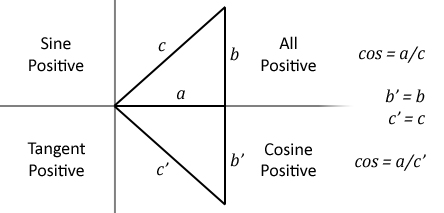# ACS

A function giving the arc cosine of its argument in radians. The permitted range of the argument is -1 to +1.

If you know the cosine of the angle, this function will tell you the angle (in radians). Unfortunately, you cannot do this with complete certainty because two angles within the range +/- PI (+/- 180 degrees) can have the same cosine. This means that one cosine has two associated angles.

The following diagram illustrates the problem:Within the four quadrants, there are two angles which have the same cosine, two with the same sine and two with the same tangent. When you are working back from the cosine, sine or tangent you don't know which of the two possible angles is correct.

By convention, ACS gives a result in the top two quadrants (0 to PI — 0 to 180 degrees) and ASN and ATN in the right-hand two quadrants (-PI/2 to +PI/2 — -90 to + 90 degrees).

In the example below, radian_angle becomes equal to the angle (in radians) whose cosine is y.

`radian_angle=ACS(y)`

You can convert the answer to degrees by using the DEG function (or multiplying by 180/PI).

`degree_angle=DEG(ACS(y))`

## Syntax

<n-var>=ACS(<numeric>)